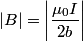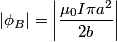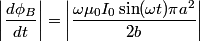## Solution to 1986 Problem 81

 We approximate the magnetic field inside the small circular wire as a constant equal to the value of the magnetic field at the center:\begin{align*}\left|B\right| = \left|\frac{\mu_0 I}{2 b}\right|\end{align*}The flux through the small wire is then\begin{align*}\left|\phi_B\right| = \left|\frac{\mu_0 I \pi a^2}{2 b}\right|\end{align*}and the time derivative of the flux is then\begin{align*}\left|\frac{d \phi_B}{dt}\right| = \left|\frac{\omega \mu_0 I_0 \sin (\omega t) \pi a^2}{2 b}\right|\end{align*...By Faraday's Law in integral form, this is equal to the induced emf in the small loop. Thus, answer (B) is correct.